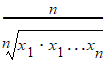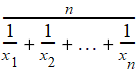# NMath User's Guide

38.6 Central Tendency (.NET, C#, CSharp, VB, Visual Basic, F#)

Measures of central tendency are measures of the location of the middle or the center of a data set. For example, the static Mean() method on class StatsFunctions computes the arithmetic mean (average) of the elements in a data set:

Code Example – C#

```double mean = StatsFunctions.Mean( data );
```

Code Example – VB

```Dim Mean As Double = StatsFunctions.Mean(MyData)
```

Median() calculates the median of the elements in a data set:

Code Example – C#

```double median = StatsFunctions.Median( data );
```

Code Example – VB

```Dim Median As Double = StatsFunctions.Median(MyData)
```

The median is the middle of the set—half the values are above the median and half are below the median. If there are an even number of elements, Median() returns the average of the middle two elements.

Mode() determines the most frequently occurring value in a data set:

Code Example – C#

```double mode = StatsFunctions.Mode( data );
```

Code Example – VB

```Dim Mode As Double = StatsFunctions.Mode(MyData)
```

GeometricMean() calculates the geometric mean.HarmonicMean() calculates the harmonic mean.TrimmedMean() calculates the mean of a data set after the specified trimming. A trimmed mean is calculated by discarding a certain percentage of the lowest and the highest values and then computing the mean of the remaining values. For example, a mean trimmed 50% is computed by discarding the lower and higher 25% of the values and taking the mean of the remaining values. TrimmedMean() takes a trimming parameter, which is a value between 0.0 and 1.0. For example, this code computes the mean trimmed 50%:

Code Example – C#

```double trimMean = StatsFunctions.TrimmedMean( data, 0.50 );
```

Code Example – VB

```Dim TrimMean As Double = StatsFunctions.TrimmedMean(MyData, 0.5)
```

The median is the mean trimmed 1.0, and the arithmetic mean is the mean trimmed 0.0.

WeightedMean() calculates the weighted average of all the elements in a data set using a given set of corresponding weights. The weighted mean is calculated asFor instance:

Code Example – C#

```var v = new DoubleVector( "-0.3 -0.03 4 2.8 -12.3 -5 3 10" );
```
```var weights = new DoubleVector( "1 2 3 4 2 1 3 4" );
```
```double weightedMean = StatsFunctions.WeightedMean( v, weights ));
```

Code Example – VB

```Dim V As New DoubleVector("-0.3 -0.03 4 2.8 -12.3 -5 3 10")
```
```Dim Weights As New DoubleVector("1 2 3 4 2 1 3 4")
```
```Dim WeightedMean As Double = StatsFunctions.WeightedMean(V,
Weights)
```

A MismatchedSizeException is raised if the number of weights does not equal the number of elements in the data set. Note that if all the weights are equal, the weighted mean is the same as the arithmetic mean.

Lastly, RMS() calculates the root mean square of the elements in a data set. RMS, sometimes called the quadratic mean, is the square root of the mean squared value.

Top

Top